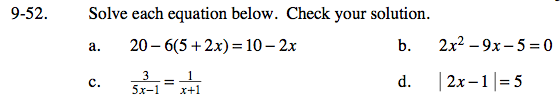### Home > GC > Chapter 9 > Lesson 9.1.5 > Problem9-52

9-52.Combine like terms after distributing.

If you find it difficult to factor, try using the Quadratic Formula.

First, multiply both sides by the lowest common denominator.

x = 2

2x− 1 = 5
2x = 6
x = 3

or

2x− 1 = − 5
2x =− 4
x = − 2